This header plots the critical line of the Riemann Zeta Function.  A complete understanding wins a \$1,000,000 prize.
 . . .Main Links Orders Post Next Page Next + 10

The 34 Convex Heptahedra and their Characteristic Polynomials
Followed by free programs you can use as you untangle the 257 convex octahedra.

 1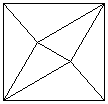x (x2+x-1) (x3-x2-9x-4) 2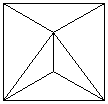x (x+1) (x+2) (x3-3x2-4x+4) 3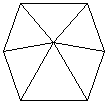(x-1)2 (x+1)2 (x+2) (x2-2x-6) 4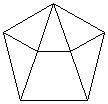(x+2) (x2-2) (x4-2x3-6x2+2x+2) 5x7-12x5-10x4+23x3+20x2-9x-6 6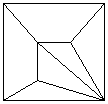x (x-1) (x+1) (x4-11x2-8x+8) 7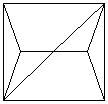x (x2-2)(x4-10x2-8x+2) 8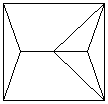x2 (x+2)2 (x3-4x2+6) 9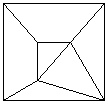(x2+x-1)2 (x3-2x2-7x+6) 10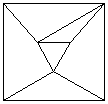x (x+2) (x5-2x4-8x3+8x2+8x-6) 11(x-1) (x+1)2 (x2-3x-1) (x3+2x2-4x-7) 12(x-1) (x+1) (x+2) (x5-2x4-8x3+8x2+11x+2) 13(x4-x3-8x2+5) (x4+x3-4x2-4x+1) 14x8-13x6-8x5+42x4+34x3-35x2-26x+4 15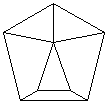(x3+2x2-x-1) (x5-2x4-8x3+9x2+11x-9) 16(x3+x2-2x-1) (x5-x4-10x3+3x2+18x-4) 17(x-1)(x+2)(x2-2)(x4-x3-8x2+4) 18x8-13x6-6x5+40x4+20x3-38x2-14x+9 19(x+1) (x7-x6-12x5+6x4+32x3-10x2-14x+4) 20(x4+x3-4x2+1) (x4-x3-8x2+5) 21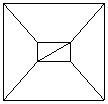(x-1) (x+1) (x2+x-1) (x4-x3-10x2+5x+9) 22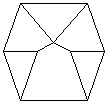(x+2) (x3-3x-1) (x5-2x4-7x3+9x2+6x-2) 23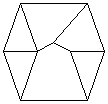(x+1) (x3+x2-2x-1) (x5-2x4-9x3+13x2+15x-8) 24(x3+x2-2x-1) (x6-x5-11x4+4x3+32x2-x+22) 25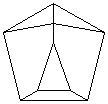(x3+2x2-x-1) (x6-2x5-9x4+11x3+24x2-12x-14) 26x (x-2) (x-1) (x+1)2 (x+2) (x3-x2-8x+4) 27(x2-x-1) (x2+x-1) (x2+3x+1) (x3-3x2-3x+8) 28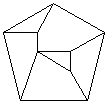x9-14x7-4x6+55x5+18x4-70x3-20x2+25x+8 29(x3+x2-2x-1) (x6-x5-11x4+8x3+20x2-7x-8) 30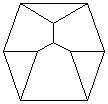(x-3) (x-1) (x+2)2 (x3-3x-1)2 31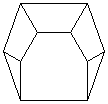(x-3) (x2-x-1) (x2+x-1) (x2+3x+1) (x3-4x-1) 32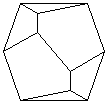x (x-3) (x-1) (x+1) (x+2) (x5+x4-7x3-5x2+10x+4) 33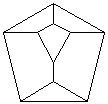(x-3) (x3+x2-2x-1)2 (x3+x2-5x-1) 34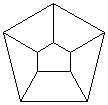(x-3)(x-1)(x2-x-1)2(x2+3x+1)2 The figures with a blue background are constructible with the Zome System. The Zome constructability of figures with a yellow background is currently unknown.

I've done an interesting study using  Matrix calculator and Factoris .  There are 7 convex hexahedra, and 34 convex heptahedra.  It is fairly easy to figure out the characteristic polynomial of each one when such tools are available.  I've started with a somewhat irregular six-sided figure.  Note that points A and C are connected.  Also, note that A and F are not connected.  To make an adjacency matrix, one merely makes a grid of ones and zeros.  Ones indicate a connection, or an adjacency.  Zeroes indicate no connection.  Since the unlying graph has six points (A B C D E and F), it has a 6x6 adjacency matrix.  You can copy the matrix in the third box, and paste it into the Matrix calculator .  Then indicate you want the characteristic polynomial.  You'll get x6 - 10x4 - 8x3 + 9x2 + 4x - 1.  If you relabel the graph in a different way (perhaps A C E F B D) and create the new adjacency matrix corresponding to this new labeling, you'll still get the same polynomial.A B C D E F A 0 1 1 0 1 0 B 1 0 0 1 1 1 C 1 0 0 1 1 0 D 0 1 1 0 0 1 E 1 1 1 0 0 1 F 0 1 0 1 1 0
0 1 1 0 1 0
1 0 0 1 1 1
1 0 0 1 1 0
0 1 1 0 0 1
1 1 1 0 0 1
0 1 0 1 1 0
The adjacency matrix of a hexahedron
Plugging x6 - 10x4 - 8x3 + 9x2 + 4x - 1 into  Factoris  gives the factorization (x3 - 2x2 - 5x + 1) (x3 + 2x2 - x - 1).  I've noticed that the characteristic polynomials of symmetric figures are more factorable than asymmetric figures.  Is there a reason for this?  I don't know, I'm just asking.  Highly symmetric graphs give highly factorable polynomials.  For example, one of many possible adjacency matrices for the  icosahedron follows.  If you paste this into Matrix calculator , then Factoris , you'll find that the icosahedron factors very nicely: x12 - 30x10 - 40x9 + 255x8 + 576x7 - 580x6 - 2640x5 - 1425x4 + 3200x3 + 5250x2 + 3000x + 625 =  (x - 5) (x + 1)5 (x2 - 5)3 .  These calculations are brutal when done by hand, but very easy with a computer.  I've factored all 34 heptahedra in the same way, and the correspondence between factorability and symmetry seems strong.

0,1,1,1,1,1,0,0,0,0,0,0
1,0,1,0,1,0,1,0,0,0,1,0
1,1,0,1,0,0,0,0,0,1,1,0
1,0,1,0,0,1,0,0,1,1,0,0
1,1,0,0,0,1,1,1,0,0,0,0
1,0,0,1,1,0,0,1,1,0,0,0
0,1,0,0,1,0,0,1,0,0,1,1
0,0,0,0,1,1,1,0,1,0,0,1
0,0,0,1,0,1,0,1,0,1,0,1
0,0,1,1,0,0,0,0,1,0,1,1
0,1,1,0,0,0,1,0,0,1,0,1
0,0,0,0,0,0,1,1,1,1,1,0
An adjacency matrix of the icosahedron

Some examples and facts --
The complete graph on m points, K(m), has the characteristic polynomial (x + 1)(m-1) (x - (m-1)).
The complete graph of m points connecting to n points,  K(m,n), has characteristic polynomial x(m+n-2)(x2 - mn).
The cube has characteristic polynomial (x-3)(x+3)(x-1)3(x+1)3
For a matrix A, the characteristic polynomial of the matrix is Determinant(A - xI).
The Cayley-Hamilton Theorem:  Any matrix A satisfies its own characteristic polynomial
The roots of a characteristic polynomial for a matrix A are also the eigenvalues for that matrix.  These numbers are called the spectrum.
The sum of roots in the spectrum for a graph is zero.
The diameter of a graph is the maximal distance between any two vertices.
The number of distinct eigenvalues in the spectrum of a graph is greater than the diameter of the graph.
In the nth power of an adjacency matrix, element aij will give the number of paths of length n between i and j.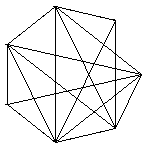-x (x + 2) (x5 - 2x4 - 12x3 - 6x2 + 10x + 6)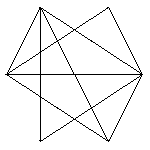(x + 1)(x5 - x4 - 9x3 - 3x2 + 10x + 4)
The above table shows two graphs which include algebraically insolvable quintics in their factorization.  Galois Theorem states that an algebraic equation is solvable if and only if its group is solvable.  Basically, this means that quintic equations and higher generally are not algebraically solvable.  They're numerically solvable, though, the second equation has roots {-1.698968584818499, -1.511052785208075, -0.4071453993213999, 1.082748288924858, 3.534418480423116}.  The sum of these is 1, their product is -4. High order equations are also solvable using series or differential equations.  I believe the polynomials above are special in some way.  Are they solvable using graphs?  That would be new to me.  Also, is there a way to find a graph, given a characteristic polynomial?  It might be an impossibly hard question, but find the graph with characteristic polynomial -(x-4) (x-1)2 (x3 + 3x2 - 1)2 .  Here's a hint : median soiree garble nebula eluant elates antrum ternes sundog. Juraj Lorinc managed to find the graph.  Here's what the hint meant: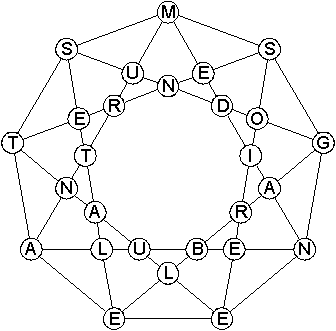The set of words here was found by Guy Jacobsen.  I haven't seen many Word Stars.  This is a (9,3) word star.  Can you make one?